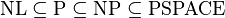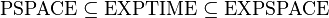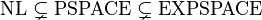# Decision problem

In recursion theory and computational complexity theory, a decision problem is a yes-or-no question on specified sets of inputs. For example, the problem "given two natural numbers x and y, does x evenly divide y?" is a decision problem. The answer can be either "yes" or "no" and depends upon the values of x and y.

Decision problems are closely related to function problems, which are the questions that can have answers that are more complex than a simple "yes" or "no:" Every decision problem can be converted into an equivalent function problem; every function problem can be converted into an equivalent decision problem. For instance, one can straightforwardly show that the decision problem, "given two numbers x and y, does x evenly divide y?" can be converted into the function problem "given two numbers x and y, what is x divided by y?" and vice versa.

A decision procedure (or decision algorithm) of a given decision problem is an effective procedure that determines the answer of the decision problem for every value of the parameters in the decision problem. For instance, a decision procedure for the decision problem "given two numbers x and y, does x evenly divide y?" would show how to determine whether x evenly divides y, given x and y for every value of "x" and "y."

When a decision problem has some decision procedure, such as in this example, the problem is said to be decidable and, otherwise, undecidable. The intuitive concept of effectiveness, which the concept of decision procedures invokes, has been considered as the formal concept, computability, (and other equivalent concepts) by the Church-Turing thesis.

The field of computational complexity theory categorizes decidable decision problems by how difficult they are to solve. "Difficult," in this sense, is described in terms of the computational resources needed by the most efficient algorithm for a certain problem. The field of computability theory, meanwhile, categorizes undecidable decision problems by Turing degree, which is a measure of the noncomputability inherent in any solution.

## Definition

A decision problem is any arbitrary yes-or-no question on specified sets of inputs. The problem "given two natural numbers x and y, does x evenly divide y?," and the problem, "In a sequence x of English alphabets, is there any occurrence of "aa"?" are examples of decision problems.

A decision procedure (or decision algorithm) of a given decision problem is an effective procedure that determines the answer of the decision problem for every value of the parameters in the decision problem. For the decision problem "given two numbers x and y, does x evenly divide y?," one example of decision procedures is taught to all school children and is called "long division."

A decision problem is called decidable if there is a decision procedure; otherwise, it is called undecidable. The intuitive notion of effectiveness procedure, which the notion of the decision procedure invokes, has been explained by the formal concept, computable functions (a function that Turing machine can compute) and other equivalent concepts such as recursive sets.

Since decision problems can be given yes-or-no answers, a decision problem can be considered as the set of inputs for which the problem returns yes. As such, a decision problem can be further considered as a set of natural numbers by associating with natural numbers inputs in the specified sets of a given decision problem in some systematic way (often by using Gödel numbering). Then formally, the decision procedure of a given decision problem can be considered in the following way. Let A be a subset of natural numbers. The decision procedure of the decision problem A is a computable function f such that, for every natural number x, f(x)=1 if x is in A; f(x)=0 if x is not in A. (Here the value 1 is considered as the answer "yes" and the value 0, as "no".) The function here is called a characteristic function of the decision problem.

## Equivalence with function problems

A function problem consists of a partial function f; the informal "problem" is to compute the values of f on the inputs for which it is defined. An example is the problem, "Given two numbers x and y, what is x divided by y?"

Every function problem can be turned into a decision problem; the decision problem is just the graph of the associated function. (The graph of a function f is the set of pairs (x,y) such that f(x) = y.) If this decision problem were decidable then the function that yields the answer of the function problem is computable.

Every decision problem can be converted into the function problem of computing the characteristic function of the set associated to the decision problem. If this function is computable then the associated decision problem is decidable.

Thus, for instance, the decision problem "given two numbers x and y, does x evenly divide y?" and the function problem "given two numbers x and y, what is x divided by y?" are equivalent in the sense that they can be converted into each other in the above way.

## Examples

Examples of decidable decision problems (considered as a subset of natural numbers) are:

• The problem whether a given number is odd (or even).
• The problem whether a given number is a prime number.
• The problem whether a given given number is in a specified finite or cofinite subset of natural numbers.

Examples of undecidable decision problems are:

• The halting problem (whether a specified Turing machine halts or runs forever).
• The busy beaver problem (determining the length of the longest halting computation among Turing machines of a specified size).
• Rice's theorem states that for all non-trivial properties of partial functions, it is undecidable whether a machine computes a partial function with that property.

## Computational complexity

Complexity theory analyzes the difficulty of computational problems in terms of many different computational resources. The same problem can be explained in terms of the necessary amounts of many different computational resources, including time, space, randomness, alternation, and other less-intuitive measures. A complexity class is the set of all of the computational problems which can be solved using a certain amount of a certain computational resource.

Examples of complexity class are:

• The complexity class P is the set of decision problems that can be solved by a (deterministic) Turing machine in polynomial time. This class corresponds to an intuitive idea of the problems which can be effectively solved in the worst cases.
• The class PSPACE is the set of decision problems that can be solved by a (deterministic) Turing machine using a polynomial amount of memory and unlimited time.

Other examples of complexity classes are NL, NP, EXPTIME, EXPSPACE. Some of the relationships between complexity classes are known. The following shows the known relationship:$\mbox{NL} \subseteq \mbox{P} \subseteq \mbox{NP} \subseteq \mbox{PSPACE}$$\mbox{PSPACE} \subseteq \mbox{EXPTIME} \subseteq \mbox{EXPSPACE}$$\mbox{NL} \subsetneq \mbox{PSPACE} \subsetneq \mbox{EXPSPACE}$

## Turing degree

The Turing degree or degree of unsolvability of a set of natural numbers measures the level of algorithmic unsolvability of the set. The concept of Turing degree is fundamental in computability theory, where sets of natural numbers are often regarded as decision problems; the Turing degree of a set tells how difficult it is to solve the decision problem associated with the set.

Two sets are Turing equivalent if they have the same level of unsolvability; each Turing degree is a collection of Turing equivalent sets, so that two sets are in different Turing degrees exactly when they are not Turing equivalent. Furthermore, the Turing degrees are partially ordered so that if the Turing degree of a set X is less than the Turing degree of a set Y then any (noncomputable) procedure that correctly decides whether numbers are in Y can be effectively converted to a procedure that correctly decides whether numbers are in X. It is in this sense that the Turing degree of a set corresponds to its level of algorithmic unsolvability.